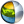Cg 3.1 Toolkit Documentation Cg / Standard Library / rsqrt

## Name

rsqrt - returns reciprocal square root of scalars and vectors.

## Synopsis

```float  rsqrt(float a);
float1 rsqrt(float1 a);
float2 rsqrt(float2 a);
float3 rsqrt(float3 a);
float4 rsqrt(float4 a);

half   rsqrt(half a);
half1  rsqrt(half1 a);
half2  rsqrt(half2 a);
half3  rsqrt(half3 a);
half4  rsqrt(half4 a);

fixed  rsqrt(fixed a);
fixed1 rsqrt(fixed1 a);
fixed2 rsqrt(fixed2 a);
fixed3 rsqrt(fixed3 a);
fixed4 rsqrt(fixed4 a);
```

## Parameters

a
Vector or scalar of which to determine the reciprocal square root.

## Description

Returns an approximation to the reciprocal square root of a.

For vectors, the returned vector contains the reciprocal square root of each element of the input vector.

The reciprocal square root of zero is ideally infinity. The square root of negative values ideally returns NaN (Not a Number).

## Reference Implementation

rsqrt is best implemented as a native reciprocal square root instruction, however rsqrt may be implemented via a pow function:

```float3 rsqrt(float3 a)
{
return pow(a, -0.5);
}
```

## Profile Support

rsqrt is supported in all profiles unless otherwise specified. rsqrt is unsupported in the fp20 profile.

In certain profiles such as vp20, rsqrt computes the absolute value of a so negative values of a will not return NaN.| Cg Toolkit | Download | Release Archive | Profiles | Reference | Books | Discussions |